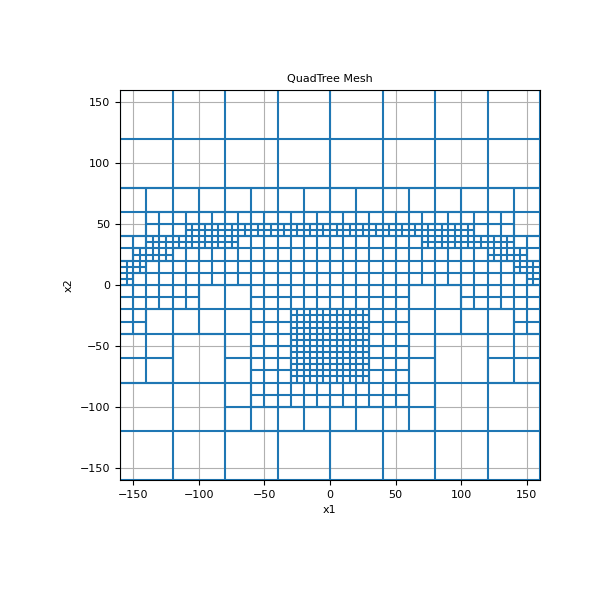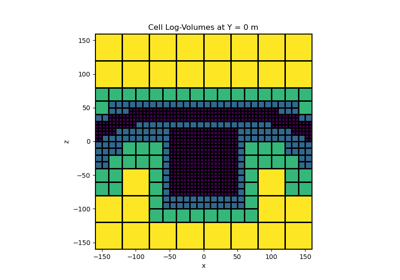# discretize.utils.refine_tree_xyz¶

Refine region within a `TreeMesh`

This function refines the specified region of a tree mesh using one of several methods. These are summarized below:

radial: refines based on radial distances from a set of xy[z] locations. Consider a tree mesh whose smallest cell size has a width of h . And octree_levels = [nc1, nc2, nc3, …] . Within a distance of nc1 x h from any of the points supplied, the smallest cell size is used. Within a distance of nc2 x (2h) , the cells will have a width of 2h . Within a distance of nc3 x (4h) , the cells will have a width of 4h . Etc…

surface: refines downward from a triangulated surface. Consider a tree mesh whose smallest cell size has a width of h. And octree_levels = [nc1, nc2, nc3, …] . Within a downward distance of nc1 x h from the topography (xy[z] ) supplied, the smallest cell size is used. The topography is triangulated if the points supplied are coarser than the cell size. No refinement is done above the topography. Within a vertical distance of nc2 x (2h) , the cells will have a width of 2h . Within a vertical distance of nc3 x (4h) , the cells will have a width of 4h . Etc…

box: refines inside the convex hull defined by the xy[z] locations. Consider a tree mesh whose smallest cell size has a width of h. And octree_levels = [nc1, nc2, nc3, …] . Within the convex hull defined by xyz , the smallest cell size is used. Within a distance of nc2 x (2h) from that convex hull, the cells will have a width of 2h . Within a distance of nc3 x (4h) , the cells will have a width of 4h . Etc…

Parameters
mesh`discretize.TreeMesh`

The tree mesh object to be refined

xyz`numpy.ndarray`

2D array of points (n, dim)

Method used to refine the mesh based on xyz locations.

• surface: Refines downward from a triangulated surface

• box: Inside limits defined by outer xy[z] locations

octree_levels`list` `of` `int`, `optional`

Minimum number of cells around points in each k octree level starting from the smallest cells size; i.e. [nc(k), nc(k-1), …] . Note that you can set entries to 0; e.g. you don’t want to discretize using the smallest cell size.

octree_levels_padding`list` `of` `int`, `optional`

Padding cells added to extend the region of refinement at each level. Used for method = surface and box. Has the form [nc(k), nc(k-1), …]

finalizebool, `optional`

Finalize the tree mesh.

min_level`int`, `optional`

Sets the largest cell size allowed in the mesh. The default (0), allows the largest cell size to be used.

max_distance`float`

Maximum refinement distance from xy[z] locations. Used if method = “surface” to reduce interpolation distance

Returns
`discretize.TreeMesh`

The refined tree mesh

Examples

Here we use the refine_tree_xyz function refine a tree mesh based on topography as well as a cluster of points.

```>>> from discretize import TreeMesh
>>> from discretize.utils import mkvc, refine_tree_xyz
>>> import matplotlib.pyplot as plt
>>> import numpy as np
```
```>>> dx = 5  # minimum cell width (base mesh cell width) in x
>>> dy = 5  # minimum cell width (base mesh cell width) in y
>>> x_length = 300.0  # domain width in x
>>> y_length = 300.0  # domain width in y
```

Compute number of base mesh cells required in x and y

```>>> nbcx = 2 ** int(np.round(np.log(x_length / dx) / np.log(2.0)))
>>> nbcy = 2 ** int(np.round(np.log(y_length / dy) / np.log(2.0)))
```

Define the base mesh

```>>> hx = [(dx, nbcx)]
>>> hy = [(dy, nbcy)]
>>> mesh = TreeMesh([hx, hy], x0="CC")
```

Refine surface topography

```>>> xx = mesh.vectorNx
>>> yy = -3 * np.exp((xx ** 2) / 100 ** 2) + 50.0
>>> pts = np.c_[mkvc(xx), mkvc(yy)]
>>> mesh = refine_tree_xyz(
...     mesh, pts, octree_levels=[2, 4], method="surface", finalize=False
... )
```

Refine mesh near points

```>>> xx = np.array([-10.0, 10.0, 10.0, -10.0])
>>> yy = np.array([-40.0, -40.0, -60.0, -60.0])
>>> pts = np.c_[mkvc(xx), mkvc(yy)]
>>> mesh = refine_tree_xyz(
...     mesh, pts, octree_levels=[4, 2], method="radial", finalize=True
... )
```

Plot the mesh

```>>> fig = plt.figure(figsize=(6, 6))## Galleries and Tutorials using `discretize.utils.refine_tree_xyz`¶